# 6-cube

6-cube
Hexeract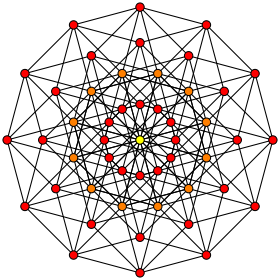Orthogonal projection
inside Petrie polygon
Orange vertices are doubled, and the center yellow has 4 vertices
TypeRegular 6-polytope
Familyhypercube
Schläfli symbol {4,34}
Coxeter-Dynkin diagrams5-faces12 {4,3,3,3}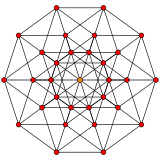4-faces60 {4,3,3}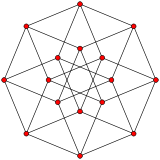Cells160 {4,3}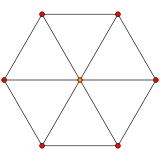Faces240 {4}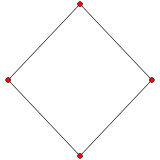Edges192
Vertices64
Vertex figure5-simplex
Petrie polygondodecagon
Coxeter groupB6, [34,4]
Dual6-orthoplexPropertiesconvex

In geometry, a 6-cube is a six-dimensional hypercube with 64 vertices, 192 edges, 240 square faces, 160 cubic cells, 60 tesseract 4-faces, and 12 5-cube 5-faces.

It has Schläfli symbol {4,34}, being composed of 3 5-cubes around each 4-face. It can be called a hexeract, a portmanteau of tesseract (the 4-cube) with hex for six (dimensions) in Greek. It can also be called a regular dodeca-6-tope or dodecapeton, being a 6-dimensional polytope constructed from 12 regular facets.

## Related polytopes

It is a part of an infinite family of polytopes, called hypercubes. The dual of a 6-cube can be called a 6-orthoplex, and is a part of the infinite family of cross-polytopes.

Applying an alternation operation, deleting alternating vertices of the 6-cube, creates another uniform polytope, called a 6-demicube, (part of an infinite family called demihypercubes), which has 12 5-demicube and 32 5-simplex facets.

## Cartesian coordinates

Cartesian coordinates for the vertices of a 6-cube centered at the origin and edge length 2 are

(±1,±1,±1,±1,±1,±1)

while the interior of the same consists of all points (x0, x1, x2, x3, x4, x5) with -1 < xi < 1.

## Images

orthographic projections
Coxeter plane B6 B5 B4
Graph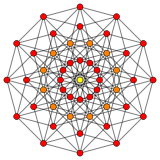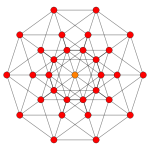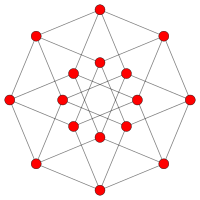Dihedral symmetry   
Coxeter plane Other B3 B2
Graph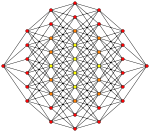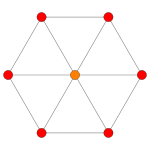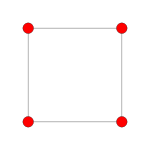Dihedral symmetry   
Coxeter plane A5 A3
Graph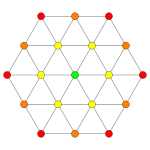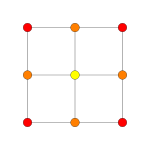Dihedral symmetry  
 3D Projections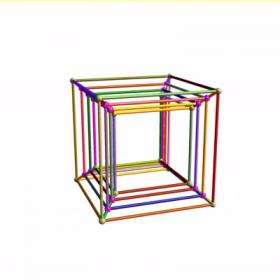6-cube 6D simple rotation through 2Pi with 6D perspective projection to 3D.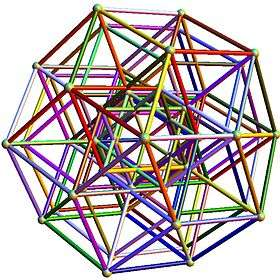Hexeract Quasicrystal structure orthographically projected to 3D using the Golden Ratio.

## Related polytopes

This polytope is one of 63 Uniform 6-polytopes generated from the B6 Coxeter plane, including the regular 6-cube or 6-orthoplex.

## References

Fundamental convex regular and uniform polytopes in dimensions 2–10
Family An Bn I2(p) / Dn E6 / E7 / E8 / E9 / E10 / F4 / G2 Hn
Regular polygon Triangle Square p-gon Hexagon Pentagon
Uniform polyhedron Tetrahedron OctahedronCube Demicube DodecahedronIcosahedron
Uniform 4-polytope 5-cell 16-cellTesseract Demitesseract 24-cell 120-cell600-cell
Uniform 5-polytope 5-simplex 5-orthoplex5-cube 5-demicube
Uniform 6-polytope 6-simplex 6-orthoplex6-cube 6-demicube 122221
Uniform 7-polytope 7-simplex 7-orthoplex7-cube 7-demicube 132231321
Uniform 8-polytope 8-simplex 8-orthoplex8-cube 8-demicube 142241421
Uniform 9-polytope 9-simplex 9-orthoplex9-cube 9-demicube
Uniform 10-polytope 10-simplex 10-orthoplex10-cube 10-demicube
Uniform n-polytope n-simplex n-orthoplexn-cube n-demicube 1k22k1k21 n-pentagonal polytope
Topics: Polytope familiesRegular polytopeList of regular polytopes and compounds